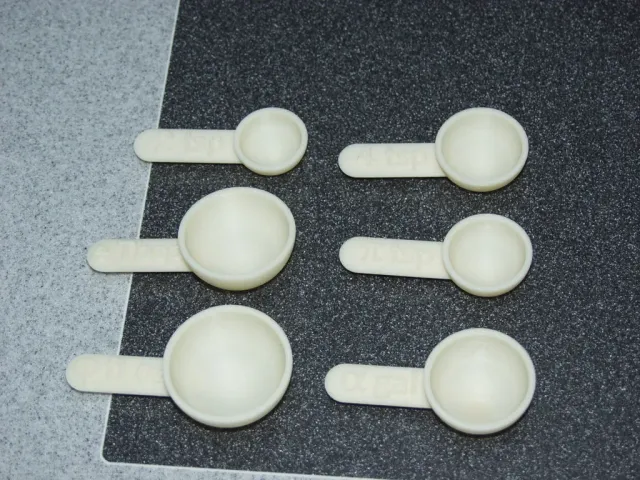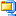# Measuring Spoons in Hard-To-Find and Useful SizesMy brother likes to drink tea, but not the kind that comes in teabags. He likes gourmet teas which have to be measured into a tea strainer for each cup, and his favorite tea requires him to measure two teaspoons of tea leaves to make a cup of tea.

It turns out that nobody makes a 2 tsp measuring spoon, so he’s always forced to measure two scoops instead of having a conveniently-sized measure which corresponds to exactly one cup’s worth of tea leaves.

This was an obvious application for a 3d printer. I designed a 2 tsp measuring spoon, using a hemisphere for the scoop so I could calculate exactly how big it needed to be. I gave it a large handle which allows it to sit exactly level on the counter, and as a final touch, embossed “2 tsp” on the handle so there’s no doubt how much it holds.

But why stop there? I occasionally have to measure 4 tsp (or 1 1/3 Tbsp) when I’m cooking, so I designed a 4 tsp measuring spoon. This lets me get in one scoop what used to take four.

Then it occurred to me: there are all kinds of useful sizes for measuring spoons which are impossible to find in the store. In particular, even the best gourmet cooking stores never seem to carry measuring spoons in increments based on mathematical and physical constants.

Hence, my set of Measuring Spoons in Hard-To-Find and Useful Sizes.

The sizes included in this set are:
2 tsp
4 tsp
pi tsp (pi is the ratio of a circle’s circumference to its diameter, see http://en.wikipedia.org/wiki/Pi )
alpha gallons (alpha is the fine structure constant, see http://en.wikipedia.org/wiki/Fine-structure_constant )
e Tbsp (e is the base of the natural logarithm, see http://en.wikipedia.org/wiki/E_(mathematical_constant) )
phi fluid ounces (phi is the golden ratio, see http://en.wikipedia.org/wiki/Golden_ratio )

Instructions
Print
Keep around the kitchen
Make puns about baking pi (optional)

For those who wonder why these measuring spoons are English rather than metric: If you’re going to make an irrational measuring spoon, it only makes sense to use an irrational measuring system, too.

Credits:

Peter L

Rate this 3D modelmeasuring-spoons-in-hard-to-find-and-useful-sizes-model_files925 KB